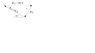# The Magnitde of Fc: Graphically

Mosaness
1. The sum of the forces FA + FB + FC = 0. The magnitudes of lFAl = 100 N and lFBl = 80N. Graphically determine the magnitude lFCl and the angle α

2. See Attachment

3. The attempts at the solution are as follows:

FBx = 80N cos 30° = 69.3 N
FBy = 80N sin 30° = 40.0 N
FAx = 100N cos 0° = 100 N
FAy = 100N sin 0° = 0N

After this, I can't figure out what to do, or if I am even on the right track.

#### Attachments

•Graph.png
3.1 KB · Views: 372

szynkasz
Sum of components of vectors in x and y direction must be zero. You can also find magnitude and angle using law of cosines

Mosaness
Sum of components of vectors in x and y direction must be zero. You can also find magnitude and angle using law of cosines

Is that something that one could do? Using the laws of cosines and sines, for situations like this?

And thank you!

azizlwl
FA + FB + FC = 0.

In components form,
FAx + FBx + FCx = 0. ...(1)
and
FAy + FBy + FCy = 0 ...(2)

Now subtitute the values in equation 1 and 2.

Homework Helper
1. The sum of the forces FA + FB + FC = 0. The magnitudes of lFAl = 100 N and lFBl = 80N. Graphically determine the magnitude lFCl and the angle α

2. See Attachment

3. The attempts at the solution are as follows:

FBx = 80N cos 30° = 69.3 N
FBy = 80N sin 30° = 40.0 N
FAx = 100N cos 0° = 100 N
FAy = 100N sin 0° = 0N

After this, I can't figure out what to do, or if I am even on the right track.

Graphically you draw a triangle of forces, with side lengths proportional to the magnitude of the forces. One side of length corresponding to Fb (80 mm for example), the other (Fa) 100 mm and the angle between them 30°. Then measure the length of the third side with a ruler and measure the angle alpha with a compass.

If you want to calculate the length of the third force, the simplest way is the law of cosines: Fc2 =Fa2+Fb2-2FaFbcos(30°). And you get the angle alpha by the law of sines:sin(alpha)/sin(30)=Fb/Fc.

But you can calculate also with the horizontal and vertical components, as you started, using that the sum of both the horizontal (x) and vertical (y) components must be zero. (Fbx+Fax+Fcx=0, Fby+Fay+Fcy=0). For that calculation, you need to take care of the signs: Fbx is negative, Fbx=-69.3 N. When you get the components Fcx and Fcy, the magnitude of Fc is Fc=√(Fcx2+Fcy2)and the angle alpha = arctan(|Fcy/Fcx|)

ehild